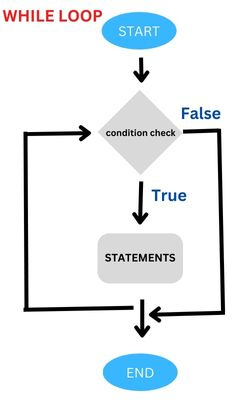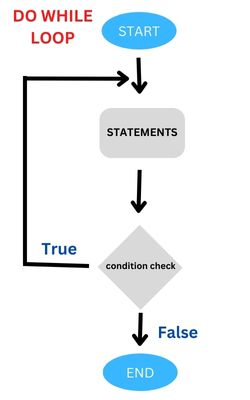# Do while loop script

``````\$i=5;
do{
echo \$i."<BR>";
\$i=\$i+1;
} while (\$i < 15);``````
Output is from 5 to 14 numbers.
Do While loop in PHP

Loops are part of the programming structure in any language. We use this at different conditions and logics inside our script. We have discussed about while loop, now we will discuss about do while loop which is little different than while loop.

## Difference in While Loop and do While loopIn while loop the condition is checked at the starting of the loop and if it is true then the code inside the loop is executed. This process is repeated till the condition becomes false. In case of do while loop the checking of condition is done at the end of the loop. So even if the condition is false, the script or statements inside the loop is executed at least once. This is the basic difference between do while loop and while loop.

Let us start with one simple script with a do while loop to understand the syntax. Here the condition is checked at the end of the loop so the code inside the loop is executed for once.
``````\$i=1;
do{
echo \$i;
echo "<br>";
} while (\$i > 1);``````
We can see in the above code that even though the \$i value is 1 which is not true as per the condition set by the loop still the script will print value of \$i once as the condition is checked at the end of the loop. To compare between these two types of loops check this code.

``````<?Php
\$i=1;
do{
echo \$i; // Out put will be there
echo "<br>";
} while (\$i > 1);
echo "<hr>";

\$i=1; // we assigned a value to the variable again here
while(\$i > 1){
echo \$i; // No output as loop will not be executed
echo "<br>";
}
?>``````

## Using break statement to stop execution of do while loop

Like other loops we can use break statement to come out of the loop. In the code below we have tried to display number from 5 to 15, but as soon as the \$i value reaches 11 the if condition becomes true and the break statement gets executed. So the loop stoops there and program execution comes out. Here is the code.
``````\$i=5;
do{
echo \$i;
echo "<br>";
\$i=\$i+1;
if(\$i>10){break;}
} while (\$i < 15);``````

## continue

continue is used within looping structures to skip the rest of the current loop iteration and continue execution at the condition evaluation and then the beginning of the next iteration.
``````\$i=5;
do{
\$i=\$i+1;
if(\$i==10){continue;} // Value 10 is not printed.
echo \$i."<BR>";
} while (\$i < 15);``````
Here output will be from 6 to 15 without printing the number 10.

Here the increment has to be before the continue, otherwise this will be an infinte loop.

## Show multiplication table

``````\$i=1;
\$j=1;
do {
echo \$i*\$j ."|";
\$j=\$j+1;
} while(\$j<=10); ``````
Output
``1|2|3|4|5|6|7|8|9|10|``

## Nested do while loops

Now we will keep one do while loop inside another outer do while loop and change \$i value from 1 to 10. We will add a line break after each inner looping.
Multiplication table for 1 to 10
``````\$i=1;
\$j=1;
do { // Outer loop
do { // Inner loop
echo \$i*\$j ."|";
\$j=\$j+1;
} while(\$j<=10); // end of inner loop
\$j=1;  // reset value to 1
\$i=\$i+1; // increment
echo "<br>"; // Line break
} while(\$i<=10) // end of outer loop ``````
Output is here
``````1|2|3|4|5|6|7|8|9|10|
2|4|6|8|10|12|14|16|18|20|
3|6|9|12|15|18|21|24|27|30|
4|8|12|16|20|24|28|32|36|40|
5|10|15|20|25|30|35|40|45|50|
6|12|18|24|30|36|42|48|54|60|
7|14|21|28|35|42|49|56|63|70|
8|16|24|32|40|48|56|64|72|80|
9|18|27|36|45|54|63|72|81|90|
10|20|30|40|50|60|70|80|90|100|``````

Subscribe to our YouTube Channel here

## Subscribe

* indicates required
Subscribe to plus2netplus2net.com

 momas 18-12-2014 good